# 基于显微放大和视觉传感的微粒辨识研究Particle Identification Based on Microscopic Amplification and Vision Sensing

• 全文下载: PDF(1148KB)    PP.73-78   DOI: 10.12677/IaE.2019.72011
• 下载量: 252  浏览量: 337

As one of the main atmospheric pollutants, airborne particulate matter has serious impacts on human health, animal plants, utensil materials and atmospheric climate. The monitoring of fine particles and ultra-clean environment is particularly important. To this end, the characteristics of atmospheric particulate matter and the tracking of its evolution process are fully studied. A microparticle identification device based on microscopic magnification and direct observation of the angle of view can be designed to observe the evolution process of particles in the atmosphere and effectively control the haze effect.

1. 引言

2. 重复性和准确性

3. 显微放大 + 视角观测

3.1. Mie散射理论基础

$\beta \left(\theta \right)=\frac{{\lambda }^{2}}{4{\text{π}}^{2}{\sigma }^{2}}\left({\omega }_{1}{\mathrm{sin}}^{2}\phi +{\omega }_{2}{\mathrm{cos}}^{2}\phi \right)\cdot {U}_{0}$

${\omega }_{1}\left(\theta \right)={|\underset{l+1}{\overset{\infty }{\sum }}\frac{2l+1}{l\left(l+1\right)}\left({a}_{l}{\text{π}}_{l}+{b}_{l}{\tau }_{l}\right)|}^{2}$

${\omega }_{2}\left(\theta \right)={|\underset{l=1}{\overset{\infty }{\sum }}\frac{2l+1}{l\left(l+1\right)}\left({a}_{l}{\tau }_{l}+{b}_{l}{\text{π}}_{l}\right)|}^{2}$

3.2. 系统主要设计Figure 1. Overall system design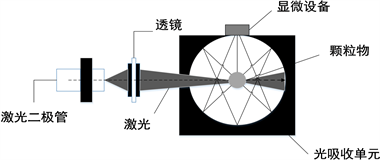Figure 2. Optical system structure design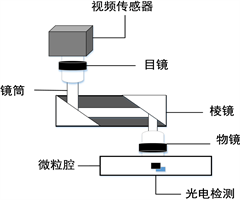Figure 3. Microsequence design

4. 仿真结果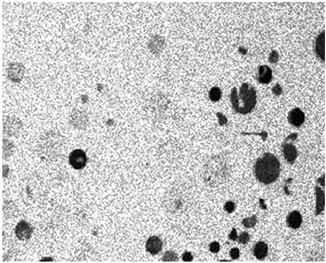Figure 4. Grayscale processed image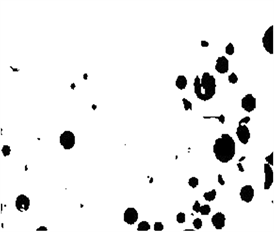Figure 5. Binarized image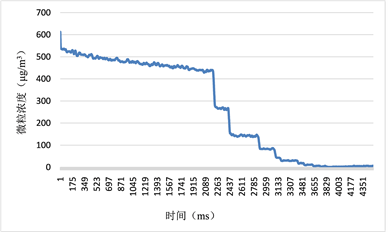Figure 6. Particle concentration analysis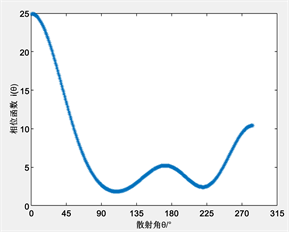Figure 7. Particle phase function with particle size of 2.5 μm

5. 结论

NOTES

*第一作者。

#通讯作者。

  Huang, R.J., Zhang, Y., Bozzetti, C., et al. (2014) High Secondary Aerosol Contribution to Particulate Pollution during Haze Events in China. Nature, 514, 218-222. https://doi.org/10.1038/nature13774  胡睿, 银燕, 陈魁, 等. 南京雾、霾期间含碳颗粒物理化特征变化分析[J]. 中国环境科学, 2017, 37(6): 2007-2015.  张小曳. 中国大气气溶胶及其气候效应的研究[J]. 地球科学进展, 2007, 22(1): 12-16.  Lighty, J.S., Veranth, J.M. and Sarofim, A.F. (2000) Combustion Aerosols: Factors Governing Their Size and Composition and Implications to Human Health. Journal of the Air & Waste Management Association, 50, 1565-1618. https://doi.org/10.1080/10473289.2000.10464197  王盾. 室内环境污染物的检测技术研究[J]. 化工管理, 2017(3): 80-81.  张福根, 荣跃龙, 周伟麟. 粒径测量及用于磨料的各种颗粒仪器[J]. 中国粉体技术, 2000(1): 26-29.  周鑫. 光散射法PM2.5传感器的性能比对及优化[J]. 环境与健康杂志, 2016, 33(8): 739-743.